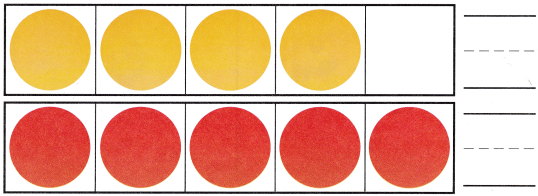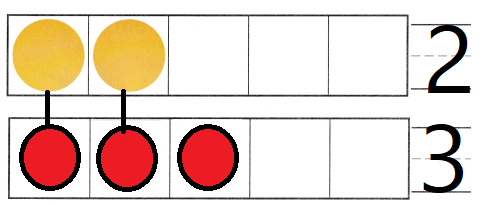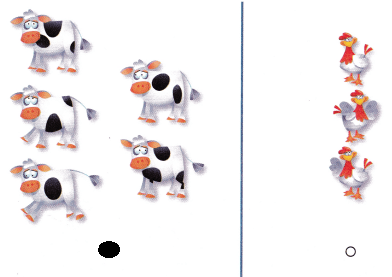# Texas Go Math Kindergarten Module 3 Assessment Answer Key

Refer to our Texas Go Math Kindergarten Answer Key Pdf to score good marks in the exams. Test yourself by practicing the problems from Texas Go Math Kindergarten Module 3 Assessment Answer Key.

## Texas Go Math Kindergarten Module 3 Assessment Answer Key

Concepts and Skills

DIRECTIONS: 1. Place a counter below each object to show the same number of objects. Draw and color each counter. Write how many objects in each row. (TEKS K.2.G) 2. Place counters as shown. Count and tell how many in each set. Write the numbers. Compare the sets by matching. Circle the number that is greater. (TEKS K.2.G)

Question 1.Explanation:
Placed a counter below each object to show the same number of objects.
Drawn and colored each counter.
3 objects in each row.

Question 2.DIRECTIONS: 3. Count and tell how many counters are in the five frame. Make a set with more counters in the five frame below. Trace and draw the counters. Write the number. Compare the sets by matching. (TEKS K.2.G) 4. Choose the correct answer. Which set has a number of animals that is greater? (TEKS K.2.G)

Question 3.Explanation:
Make a set with more counters than the yellow five frame
and written the numbers

Question 4.